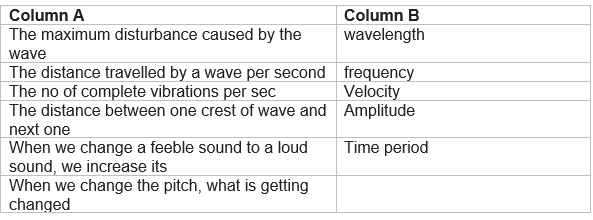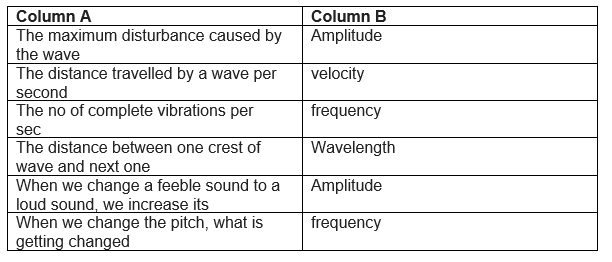# Extra questions for Class 9 Sound

Given below are the Class 9 Science Extra Questions for Sound
(a) Match thec column

## Match the column

Question 1Question 2 Name two animals which can produce infrasonic waves.

Hippopotamus and whale

Question 3
What is reverberation?

Reverberation is defined as persistence of sound after the source has stopped emitting sound.
This is due to multiple reflections of sound waves.

Question 4
What is echo?

The sound waves produced bounce back or gets reflected from the mountain or buildings and come to our ears, this reflected sound is known as Echo. To hear echo, the barrier reflecting the sound should be least at a distance of 17 meters.

Question 5
What is infrasonic? Give an example.

Sound having frequency less than 20Hz is known as infrasonic sound or infrasonic.
Waves produced during earthquake are infrasonic.

Question 6
Give examples of organisms which can hear infrasonic?

Whales and elephants can produce and hear infrasonic sound.

Question 7
What are infrasonic and ultrasonic sounds?

Infrasonic sound has frequency less than 20 Hz and ultrasonic sound has frequency higher than 20 kHz.

Question 8
What is the audible range of human ear?

The audible range of human ear is 20 Hz to 20 kHz.

Question 9
Why do we hear sound of an approaching truck before the truck reaches us?

This is because velocity of sound is much greater than the velocity of truck

Question 10
What is the frequency of wave with time period 0.025 s?

Frequency (f) =1/Time period
=1/0.025
= 40Hz
Therefore, frequency of the wave = 40 Hz.

Question 11
A baby recognizes her mother by her voice. Name the characteristic of sound involved

The characteristic of sound involved in uniqueness of the sound is quality of sound or timber.

Question 12.
A person hears an echo from the top of a tower, 2.2 sec after the sound is produced. How far away is the tower from the person? Speed of the sound in air is 332m/s?

Distance travelled by sound= 332 * 2.2=730.4 m
Distance of tower from person= (Distance travelled by sound)/2 = 730.4/2=365.2 m

Question 13.
How can multiple echoes of a single sound be produced? Explain?
Question 14.
How temperature affects the velocity of sound?
Question 15.
When a sound is reflected from a distant object, an echo is produced and heard on a winter night. Will you hear the echo of the same sound on a summer day if the distance between reflecting surface and the source of sound remains the same? Justify your answer?
Question 16.
Why are the walls and roof of an auditorium covered with sound absorbent materials?
Question 17.
Define reverberation. What arrangements should be made in an auditorium to control excessive reverberation?
Question 18.
Determine the minimum distance between the listener and reflector for an echo to be heard dintinctively of a sound propagating with a speed v m/s?

The perception of a sound usually endures in memory for only 0.1 seconds so, minimum time for echo = 0.1 sec
Let d be the minimum distance
Now sound have to travel, from listener to reflector then to listener
so total distance travelled = 2d
now,
distance = speed x time = v * .1
So,
2d=v/10
or
d=v/20

Question 19.
Name the sound of the following frequencies:
(a)5Hz
(b)50Hz
(c)50000Hz
(d)50kHz

(a) infrasonic sound
(b)audible sound
(c) Ultrasonic
(d) Ultrasonic

Question 20.
What is the frequency of a wave whose time period is 0.05s?

Frequency (f) =1/Time period
=1/0.05
= 20Hz
Therefore, frequency of the wave = 20 Hz.

Question 21.
A ship is stationary is at a distance of 2800m from the sea-bed. The ship sends an ultrasound signal to the sea-bed and its echo is heard after 4s. Find the speed of sound in water?
Question 22.
How do the frequency and amplitudes affect musical sound?
Question 23. A hospital uses an ultrasonic scanner to locate tumors in a tissue. What is the wavelength of ultrasound in a tissue in which the speed of ultrasound is 1.5km/sec2 ? The operating frequency of the scanner is 4.0 MHz?
Question 24.
A ship sends out ultrasound that returns from the sea-bed and is detected after 3.22sec. If the speed of ultrasound in sea water is 1530m/s, calculate the distance of the sea-bed from the ship?
Question 25
What is SONAR? For what it is used?

SONAR is Sound Navigation And Ranging. It is a technique used to measure the depth of the sea, locate the sunken ships or icebergs and submarines.

Question 26.
How bats can detect their prey?
Question 27.
A body is vibrating 6000times in one minute. If the velocity of sound in air is 360m/s, Find:
(a)Frequency of the vibration in Hz
(b)Wavelength of sound produced
Question 28.
Explain with the help of a diagram the working of SONAR?
Question 29.
State any two characteristics of a wave motion?
Question 30.
Explain how the human ear works?
Question 31.
Give two practical applications of reflection of sound waves?
Question 32.
Briefly mention three uses of ultrasound in the field of medicine?
Question 33.
Distinguish between transverse waves and longitudinal waves?
Question 34.
When vertically upward jerk is given to a string, transverse waves are formed? Give three reasons?
Question 35
A body is vibrating 12000 times in one minute. If the velocity of sound in air is 360 m/s, find:
(a) Frequency of vibration in hertz,
(b) Wavelength of the wave produced.

(a) Frequency of vibration in hertz
Given,
Number of vibration in one minute = 12000
Number of vibrations in one sec =12000/60
=200Hz
Therefore, Frequency, f = 200 Hz
(b) Wavelength 2f the wave produced
Given,
Velocity of speed in air, v = 360 m/s
Frequency, f = 200 Hz
v = f λ
or λ=v/f
=360 /200 = 1.8m

## Summary

This Class 9 Extra questions for Sound is prepared keeping in mind the latest syllabus of CBSE . This has been designed in a way to improve the academic performance of the students. If you find mistakes , please do provide the feedback on the mail.